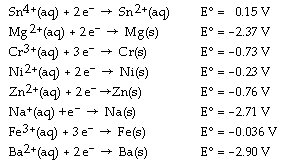# Problem: Determine which of the following pairs of reactants will result in a spontaneous reaction at 25°C.a. Zn(s) + Na+(aq)b. Fe(s) + Ba2+(aq)c. Cr3+(aq) + Ni(s)d. Sn4+(aq) + Mg(s)e. None of the pairs will react.

🤓 Based on our data, we think this question is relevant for Professor Rothberg's class at UR.

###### FREE Expert Solution###### Problem Details

Determine which of the following pairs of reactants will result in a spontaneous reaction at 25°C.

a. Zn(s) + Na+(aq)

b. Fe(s) + Ba2+(aq)

c. Cr3+(aq) + Ni(s)

d. Sn4+(aq) + Mg(s)

e. None of the pairs will react.